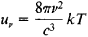# Rayleigh-Jeans Law

(redirected from Rayleigh-Jeans Limit)

## Rayleigh-Jeans law

[¦rā·lē ′jēnz ‚lȯ]
(statistical mechanics)
A law giving the intensity of radiation emitted by a blackbody within a narrow band of wavelengths; it states that this intensity is proportional to the temperature divided by the fourth power of the wavelength; it is a good approximation to the experimentally verified Planck radiation formula only at long wavelengths.

## Rayleigh-Jeans Law

a law expressing the energy distribution in the spectrum of a blackbody as a function of temperature. The Rayleigh-Jeans law may be written in the formwhere uv is the radiation density corresponding to the frequency v, c is the speed of light, T is the absolute temperature, and k is the Boltzmann constant.

The Rayleigh-Jeans law was derived in 1900 by Lord Rayleigh from classical concepts of the uniform distribution of energy with respect to degrees of freedom. In work conducted between 1905 and 1909, J. Jeans applied the methods of classical statistical mechanics to standing waves in a cavity and arrived at the same equation as Rayleigh.

The Rayleigh-Jeans law of radiation is in good agreement with experiment only for small v—that is, for long wavelengths. According to the law, as v increases, the radiant energy should increase without bound. In the far ultraviolet and in still shorter-wavelength regions of the spectrum, the density of radiant energy should reach extremely large values, a situation called the ultraviolet catastrophe. This prediction, however, is inconsistent with experiment. A blackbody energy distribution valid for the entire spectrum can be obtained only on the basis of quantum concepts (see PLANCK’S RADIATION LAW). The Rayleigh-Jeans law is a special case of Planck’s law for small v and can be used instead of Planck’s law when radiation at sufficiently long wavelengths is being considered and when high accuracy of calculation is not required.

### REFERENCES

Planck, M. Teoriia teplovogo izlucheniia. Moscow-Leningrad, 1935. (Translated from German.)
Shpol’skii, E. W. Atomnaiafizika, 5th ed., vol. 1. Moscow, 1963.
Site: Follow: Share:
Open / Close